# 个人博客转至：tybai.com# 【机器学习】算法原理详细推导与实现(二):逻辑回归

## logistic函数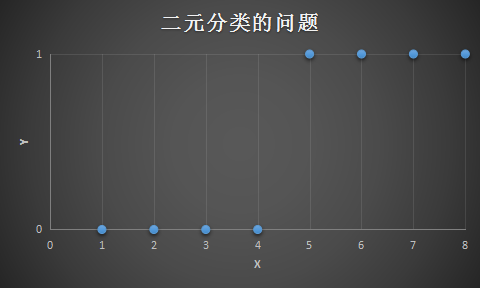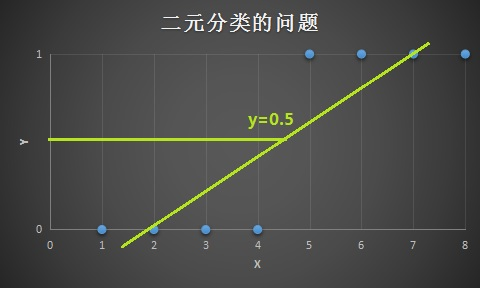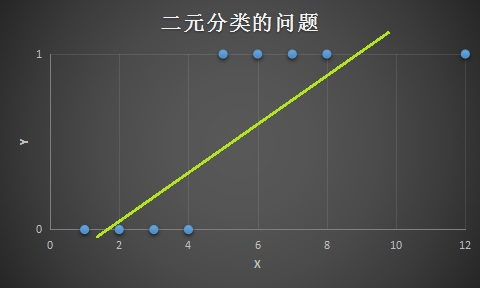$g(z)=\frac{1}{1+e^{-z}}$

$h_\theta(x)=g(\theta^Tx)=\frac{1}{1+e^{-\theta^Tx}}$

$g(z)$ 被称为 sigmoid函数 ，也通常被称为 logistic函数，它的函数图像是：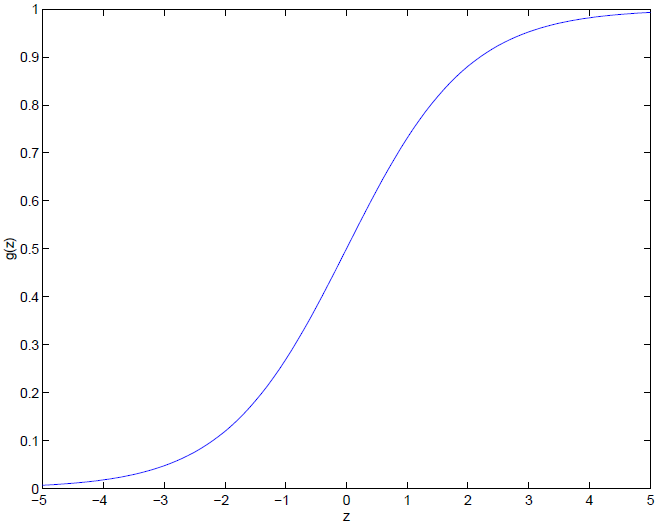$z$ 变得非常小的时候，$g(x)$ 会趋向于0，当$z$变得非常大的时候，$g(x)$ 会趋向于1，它和纵轴相较于0.5。

## 逻辑回归

$P(y=1|x;\theta)=h_\theta(x)$

$P(y=0|x;\theta)=1-h_\theta(x)$

$P(y|x;\theta)=(h_\theta(x))^y(1-h_\theta(x))^{1-y}$

$\begin{split} L(\theta)&=P(\vec{y}|X;\theta) \\ &=\prod_{i=1}^mP(y^{(i)}|x^{(i)};\theta) \\ &=\prod_{i=1}^m(h_\theta(x^{(i)}))^{y^{(i)}}(1-h_\theta(x^{(i)}))^{1-{y^{(i)}}} \end{split}$

$\begin{split} l(\theta)&=logL(\theta) \\ &=\sum_{i=1}^m{y^{(i)}logh(x^{(i)})+(1-y^{(i)})log(1-h(x^{(i)}))} \end{split}$

$\theta:=\theta-\alpha\frac{\partial J(\theta)}{\partial\theta}=>\theta:=\theta-\alpha\nabla_\theta J(\theta)$

$\theta:=\theta+\alpha\nabla_\theta l(\theta)=>\theta:=\theta+\alpha\frac{\partial l(\theta)}{\partial\theta}$

$\begin{split} \frac{\partial l(\theta)}{\partial\theta_j}&=(y\frac{1}{g(\theta^Tx)}-(1-y)\frac{1}{1-g(\theta^Tx)})\frac{\partial}{\partial\theta_j}g(\theta^Tx) \\ &=(y\frac{1}{g(\theta^Tx)}-(1-y)\frac{1}{1-g(\theta^Tx)})g(\theta^Tx)(1-g(\theta^Tx))\frac{\partial}{\partial\theta_j}\theta^Tx \\ &=(y(1-g(\theta^Tx))-(1-y)g(\theta^Tx))x_j \\ &=(y-g(\theta^Tx))x_j \\ &=(y-h_{\theta}(x))x_j \end{split}$

$\frac{\partial l(\theta)}{\partial\theta_j}=\sum_{i=1}^m{(y-h_{\theta}(x))x_j}$

$\theta_j:=\theta_j+\sum_{i=1}^m{(y^{(i)}-h_{\theta}(x^{(i)}))x^{(i)}_j}$

## 鸢尾花分类

5.1 3.5 0
4.9 3.0 0
4.7 3.2 0
7.0 3.2 1
6.4 3.2 1
... ... ...

0 山鸢尾（setosa）
1 变色鸢尾（versicolor）
2 维吉尼亚鸢尾（virginica）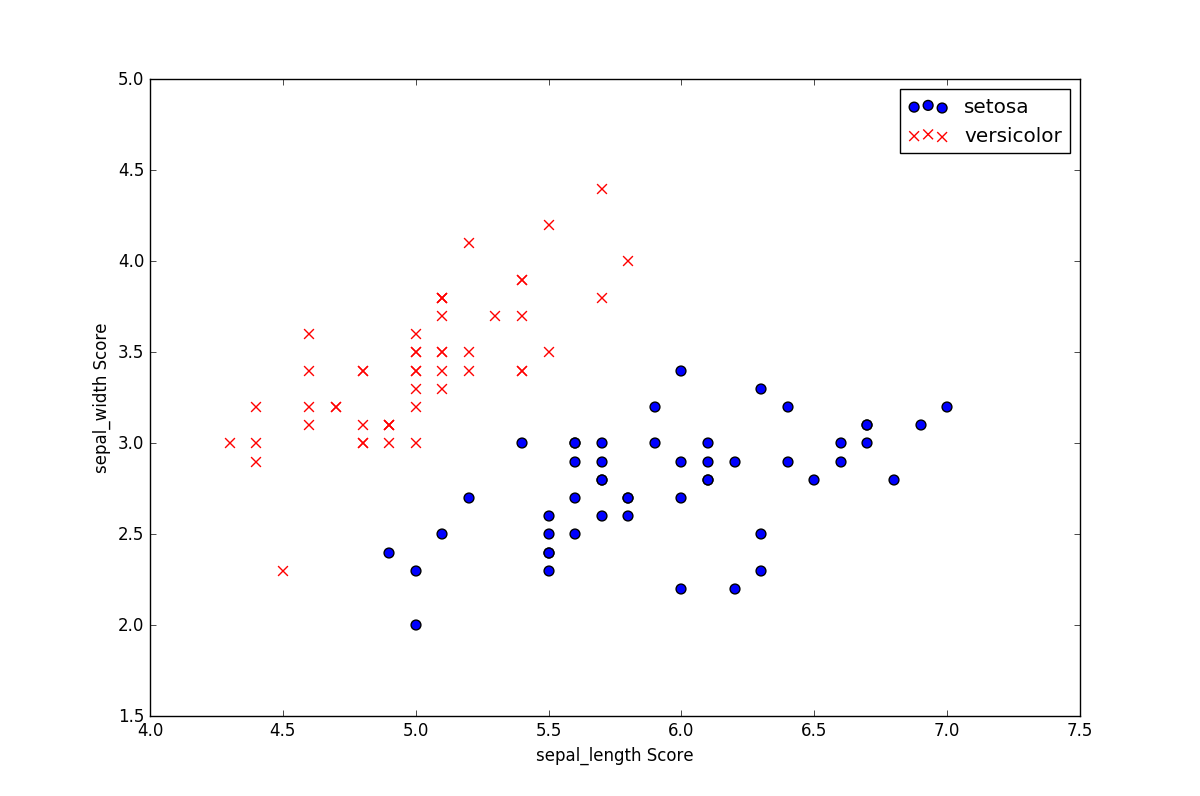# 损失函数
def computeCost(theta, X, y):
theta = np.matrix(theta)
X = np.matrix(X)
y = np.matrix(y)
first = np.multiply(-y, np.log(sigmoid(X * theta.T)))
second = np.multiply((1 - y), np.log(1 - sigmoid(X * theta.T)))
return np.sum(first - second) / (len(X))

# 梯度下降
def gradient(theta, X, y):
theta = np.matrix(theta)
X = np.matrix(X)
y = np.matrix(y)

parameters = int(theta.ravel().shape)
grad = np.zeros(parameters)

error = sigmoid(X * theta.T) - y

for i in range(parameters):
term = np.multiply(error, X[:, i])
grad[i] = np.sum(term) / len(X)

return grad

accuracy = 99%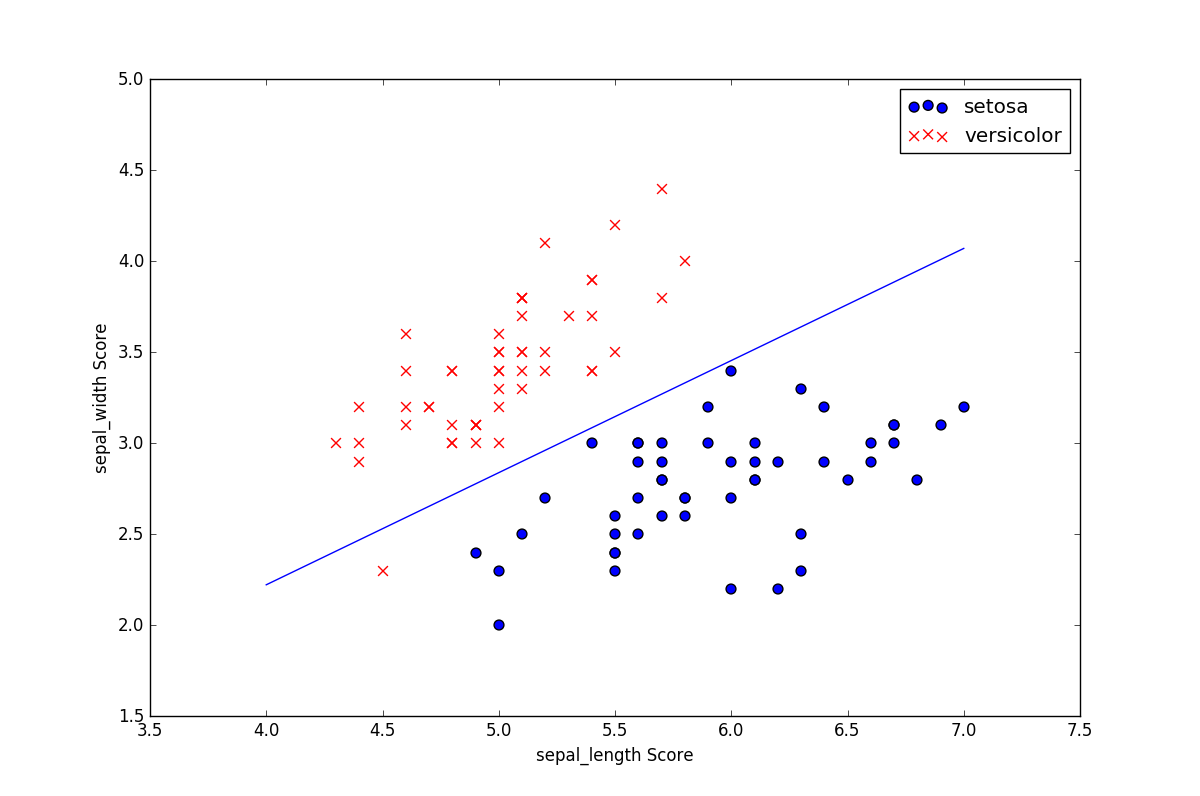posted on 2019-06-25 10:19 TTyb 阅读(...) 评论(...) 编辑 收藏

### 导航